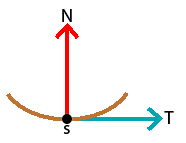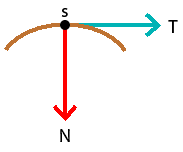## The curvature and torsion: how to distinguish the shape of a curve### The Curvature (plane case)

Let $$f:\, I\rightarrow\mathbb{R}^{2}$$ be a differentiable parametrized curve. For each $$t\in I$$ with $$f'(t) \neq 0$$, there is a unique line that passes through point $$f(t)$$ and has the direction of the vector $$f'(t)$$. This is the tangent line to the curve $$f$$ at $$t$$. In the study of curves, it is usual (and convenient) to assume the existence of the tangent at every point of the curve:

Definition: A differentiable parametrized curve $$f:\, I\rightarrow\mathbb{R}^{2}$$ is regular if $$f'(t) \neq 0$$ for every $$t \in I$$.
The arc length of a regular parametrized curve $$f:\, I\rightarrow\mathbb{R}^{2}$$, from point $$t_{0} \in I$$, is the function $s(t)=\int_{t_{0}}^{t}\left|f'(t)\right|dt.$

Note that $$s'(t)=\left|f'(t)\right|$$.

A regular parametrized curve is said to be parametrized by arc length if $$\left|f'(t)\right|=1$$ for every $$t \in I$$.

Assuming that a curve $$f$$ determines the position of a certain particle, the fact that the curve is parametrized by arc length means that the particle is moving at constant speed $$1$$.

Furthermore: the tangent vector $$T=\left(T_{1},T_{2}\right)$$ to the curve at a certain $$t$$ is given by $$f'(t)$$ and the normal vector $$N=\left(N_{1},N_{2}\right)$$ is given by $\frac{f''(t)}{\left|f''(t)\right|}.$

The definition of curvature that we present below is only valid for curves parametrized by arc length. This restriction is not really significative: given any smooth curve, it is possible to determine a differentiable curve parametrized by arc length which has the same trace as the given smooth curve.

Definition: Let $$f:\, I\rightarrow\mathbb{R}^{2}$$ be a curve parametrized by arc length $$s \in I$$. The curvature (with sign) of $$f$$ is given by $k(s)=§\left|f'(s)\right|$ where $$§$$ is defined in the following way
Value of $$§$$ Condition "Scheme"
$$§=1$$ $$T_{1}\times N_{2}-T_{2}\times N_{1} >0$$$$§=-1$$ $$T_{1}\times N_{2}-T_{2}\times N_{1} <0$$